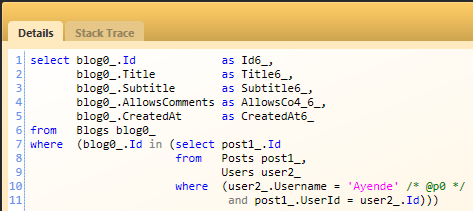## NHibernate Queries - Examples

time to read 8 min | 1518 words

Today was the first day of my NHibernate course, and I think that it might be good to point out a few of the samples that we worked with. Those are pretty basic NHibernate queries, but they are probably going to be useful for beginners.

Let us take my usual Blog model, and see what kind of queries (and results) we can come up with:

Let us find a blog by its identifier:

`var blog = s.Get<Blog>(1);`

Which results in:We can also try:

Which would result in… absolutely no SQL queries. You can look at a more deep discussion of that here.

Now, let us try to search by a property:

`var blogs = s.CreateCriteria<Blog>()    .Add(Restrictions.Eq("Title", "Ayende @ Rahien"))    .List<Blog>();`

Which results in:

If we try to make the same with HQL, it would look:

`var blogs = s.CreateQuery("from Blog b where b.Title = :title")    .SetParameter("title","Ayende @ Rahien")    .List<Blog>();`

Which results in slight different SQL than using the criteria:

What about trying a more complex conditional? Let us try to see comparing two properties:

`var blogs = s.CreateCriteria<Blog>()    .Add(Restrictions.Eq("Title","Ayende @ Rahien"))    .Add(Restrictions.Eq("Subtitle", "Send me a patch for that"))    .List<Blog>();`

Which results in:

Let us do that again, but using two properties using an OR:

`var blogs = s.CreateCriteria<Blog>()    .Add(Restrictions.Disjunction()             .Add(Restrictions.Eq("Title", "Ayende @ Rahien"))             .Add(Restrictions.Eq("Subtitle", "Send me a patch for that")))    .List<Blog>();`

Which would result in:

We can also execute the same SQL using the following syntax:

`var blogs = s.CreateCriteria<Blog>()    .Add(            Restrictions.Eq("Title", "Ayende @ Rahien") ||             Restrictions.Eq("Subtitle", "Send me a patch for that")        )    .List<Blog>();`

Doing the same using HQL would be:

`var blogs = s.CreateQuery("from Blog b where b.Title = :title and b.Subtitle = :subtitle")    .SetParameter("title","Ayende @ Rahien")    .SetParameter("subtitle", "Send me a patch for that")    .List<Blog>();`

Which results in:

And changing that to an OR is pretty self explanatory :-)

`var blogs = s.CreateQuery("from Blog b where b.Title = :title or b.Subtitle = :subtitle")    .SetParameter("title","Ayende @ Rahien")    .SetParameter("subtitle", "Send me a patch for that")    .List<Blog>();`

Giving us:

Let us try something a bit more complex, finding a blog by a post title:

`var blogs = s.CreateCriteria<Blog>()    .CreateCriteria("Posts")    .Add(Restrictions.Eq("Title","NHibernate Rocks"))    .List<Blog>();`

That gives us:

You will note that we force a load of the Posts collection. We can try something else, though:

`var blogs = s.CreateCriteria<Blog>()    .Add(Subqueries.PropertyIn("id",        DetachedCriteria.For<Post>()            .Add(Restrictions.Eq("Title","NHibernate Rocks"))            .SetProjection(Projections.Property("Blog.id"))        ))    .List<Blog>();`

Which would give us the same result, but without loading the Posts collection:

This is a pretty common example of changing the way that we compute complex conditionals when we want to avoid wide result sets.

Let us do the same with HQL:

`var blogs = s.CreateQuery("from Blog b join b.Posts p where p.Title = :title")    .SetParameter("title", "NHibernate Rocks")    .List<Blog>();`

Which would result:

We have the same issue as with the first Criteria API, and we can resolve it in the same way:

`var blogs = s.CreateQuery("from Blog b where b.id in (from Post p where p.Title = :title)")    .SetParameter("title", "NHibernate Rocks")    .List<Blog>();`

And the same result as in the Criteria API show us:

And the final test, let us try to find a blog that has a post posted by a specific user:

`var blogs = s.CreateCriteria<Blog>()    .Add(Subqueries.PropertyIn("id",        DetachedCriteria.For<Post>()            .SetProjection(Projections.Property("Blog.id"))            .CreateCriteria("User")            .Add(Restrictions.Eq("Username","Ayende"))        ))    .List<Blog>();`

And this give us:

The same thing with HQL will give us:

`var blogs = s.CreateQuery("from Blog b where b.id in (from Post p where p.User.Username = :user)")    .SetParameter("user","Ayende")    .List<Blog>();`

And that results: